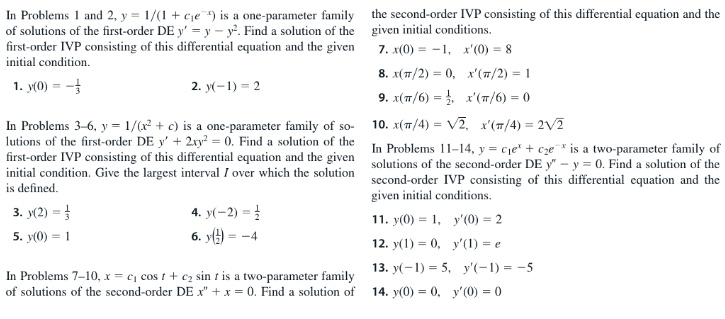# In Problems 1 and 2, y = 1/(1 + cje ) is a one-parameter family of solutions of the first-order DE y' = y – y. Find a solution of the first-order IVP consisting of this differential equation and the given the second-order IVP consisting of this differential equation and the given initial conditions. 7. x(0) = -1, x'(0) = 8 initial condition. 8. x(7/2) = 0, x'(7/2) = 1 1. y(0) = - 2. y(-1) = 2 (π/ )-0 9. x(π/6)-, In Problems 3-6, y = 1/(x + c) is a one-parameter family of so- lutions of the first-order DE y' + 2ry = 0. Find a solution of the 10. x(7/4) = V2, x'(T/4) = 2V2 In Problems 11–14, y = cje' + cze* is a two-parameter family of first-order IVP consisting of this differential equation and the given initial condition. Give the largest interval I over which the solution is defined. solutions of the second-order DE y" - y = 0. Find a solution of the second-order IVP consisting of this differential cquation and the given initial conditions. 3. y(2) = 4. y(-2) = 1 11. y(0) = 1, y'(0) = 2 6. y() = 5. y(0) = 1 = -4 12. y(1) = 0, y'(1) = e 13. y(-1) = 5, y'(-1) = -5 In Problems 7-10, x = e, cos t+ c2 sin t is a two-parameter family of solutions of the second-order DE x" +x = 0. Find a solution of 14. y(0) = 0, y'(0) = 0

Question

#7help_outlineImage TranscriptioncloseIn Problems 1 and 2, y = 1/(1 + cje ) is a one-parameter family of solutions of the first-order DE y' = y – y. Find a solution of the first-order IVP consisting of this differential equation and the given the second-order IVP consisting of this differential equation and the given initial conditions. 7. x(0) = -1, x'(0) = 8 initial condition. 8. x(7/2) = 0, x'(7/2) = 1 1. y(0) = - 2. y(-1) = 2 (π/ )-0 9. x(π/6)-, In Problems 3-6, y = 1/(x + c) is a one-parameter family of so- lutions of the first-order DE y' + 2ry = 0. Find a solution of the 10. x(7/4) = V2, x'(T/4) = 2V2 In Problems 11–14, y = cje' + cze* is a two-parameter family of first-order IVP consisting of this differential equation and the given initial condition. Give the largest interval I over which the solution is defined. solutions of the second-order DE y" - y = 0. Find a solution of the second-order IVP consisting of this differential cquation and the given initial conditions. 3. y(2) = 4. y(-2) = 1 11. y(0) = 1, y'(0) = 2 6. y() = 5. y(0) = 1 = -4 12. y(1) = 0, y'(1) = e 13. y(-1) = 5, y'(-1) = -5 In Problems 7-10, x = e, cos t+ c2 sin t is a two-parameter family of solutions of the second-order DE x" +x = 0. Find a solution of 14. y(0) = 0, y'(0) = 0 fullscreen

1 Rating

### Want to see this answer and more?

Experts are waiting 24/7 to provide step-by-step solutions in as fast as 30 minutes!*

*Response times may vary by subject and question complexity. Median response time is 34 minutes for paid subscribers and may be longer for promotional offers.
Tagged in
Math
Calculus

### Other